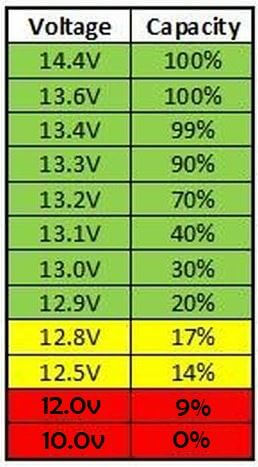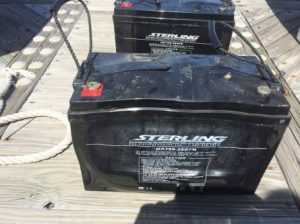# MPPT charge controllers: A complete but quick overview

MPPT charge controllers are expensive, but they are the best charge controller technology on the market. In this article, I’ll answer some of the FAQs about these devices. If there are any questions I haven’t answered, please feel free to ask in the comment section.

## What are MPPT charge controllers and what do they do?

MPPT charge controllers – also called Maximum Power Point Trackers – are efficient DC-DC converters used in solar systems to connect solar panels to batteries and DC loads.

MPPT charge controllers regulate the voltage and the current from the solar array to match the requirements of a charging battery and consequently protect it.

The main advantage of  MPPT charge controllers is that while protecting the battery, they manage to optimize the output power of the solar array and minimize energy losses.

These electronic devices are categorized by their Output Current (in Amps) and come in different sizes. The higher the amperage rating on an MPPT charge controller, the more Amps (current) it is able to put out.

They are also limited by their maximum input and output voltages. For example, consider this MPPT charge controller from Victron.

• It has an Amperage rating of 30A: which means it will not put out more than 30 Amps of current.
• It has a Maximum Input Voltage of 100V: meaning that the maximum voltage of the solar array connected to it has to be lower than 100V.
• It is designed to work with 12V and 24V battery banks: which means it will not work with 36V or 48V battery banks.

## How do MPPT charge controllers work?

MPPT solar charge controllers have 2 main circuits, so they basically perform 2 operations:

1. Maximize the power output of the solar array through Maximum Power Point Tracking technology.
2. Decrease the voltage of the solar array to match the voltage of the battery while increasing the current by the same ratio.

Let’s see what this means exactly.

### Maximum power point tracking

On the input circuit, the MPPT charge controller makes sure that the solar array is producing as much power as possible.

This is done by finding the voltage for which the power produced by the solar panel is at its peak. This particular voltage is called the Maximum Power Voltage (Vmp or Vmpp).IV characteristic of a solar cell

At this voltage, the solar array produces the Maximum Power Current (Imp or Impp). The product of the Vmp and Imp gives us the Maximum Power (Pmax or Pmpp).

Pmax = Vmp x Imp

Once the MPPT finds the Vmp, it makes sure that the voltage and current are maintained at that particular point.

If there are any changes in temperature or solar irradiance (sunlight hitting the solar panels), the charge controller finds a new Vmp.

### Matching battery voltage

On the output circuit, the MPPT charge controller lowers the output voltage of the solar array to match that of the battery bank. And although it decreases the voltage, it also increases the current by the same ratio. This power transformation ensures that there are no losses in power.

For example: Consider a 100W-12V solar panel charging a 12V battery.

The voltage of the panel is actually a little bit higher than 12 Volts.

When the sun is up, the actual voltage of the panel is somewhere around 17V – 19V.Spec sheet of a 100W solar panel from Renogy

On the other hand, the voltage that the 12V battery requires to charge varies from 10V to 14.4V depending on the state of charge of the battery and its chemistry.If this solar panel is directly connected to the battery, the battery will eventually experience overvoltage, which leads to all sorts of irreversible damage. To prevent that from happening, the MPPT limits that voltage to an appropriate value at all times.

Also, when the battery is almost charged, the MPPT regulates the power from the solar panel to prevent battery overcharging.

At a high state of charge, if the power from the solar panel is left unregulated and overcharging occurs, the battery will end up overheating and eventually failing prematurely.Credit.

MPPT charge controllers prevent these problems by lowering the voltage to an acceptable charging voltage and limiting the power delivered to the battery when it’s at a high state of charge.

Following our example, here is the power transformation carried out by the MPPT:

Power = Voltage x Current

100W= 18.6V x 5.38A

When a 12V battery is at 0% state of charge (depleted), its voltage is around 10 Volts. The MPPT decreases the output voltage from the solar panel to around 10.5V (just above 10 Volts) and increases the current by the same ratio to maintain maximum power:

Transformed Current = 100W ÷ 10.5V

Transformed Current = 9.5 Amps

But like any electronic device, MPPT charge controllers are not 100% efficient. a 1% – 5% loss in power is within the norms.

## Do I need an MPPT charge controller?

In some cases, the answer is yes, but you do not necessarily always need an MPPT charge controller for your system. However, if you want your solar setup to last as long as it should, you do need a solar charge controller.

As mentioned above, without a solar charge controller your batteries are at risk of being damaged. Even if you’re using a small solar panel (5W – 10W) to trickle charge your battery, you will still need a solar charge controller.

With small solar panels, a PWM charge controller can be used to regulate the voltage and protect the battery.

However, with bigger solar installations where lowering the voltage without compensating in current can cause a significant loss in power, MPPT solar charge controllers are the best option.

## What size MPPT charge controller do I need?

To select the right MPPT charge controller, you need to calculate how much current you’ll need the MPPT to put out.

To do that, you can use this equation:

MPPT amperage rating = (System Wattage) / (Lowest Battery Charging Voltage)

MPPT charge controllers also have an input voltage limit, so while choosing one, you also have to account for the maximum voltage from your solar array.

You can do this correctly by checking out this article on how to size an MPPT charge controller, or you can use our MPPT sizing calculatorRenewableWise’s MPPT size calculator

By following a few steps, this MPPT calculator will calculate the specs of the charge controller you need and give you a couple of options.